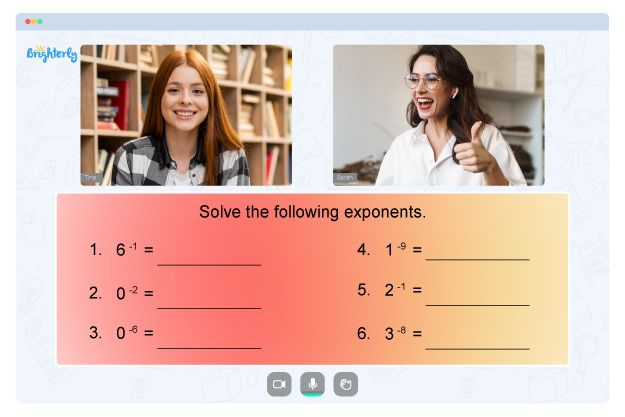# Properties of Logarithms Worksheets

A logarithmic properties worksheet is a valuable educational resource that helps students understand and master the fundamental concepts and rules that govern logarithms. Logarithmic properties worksheets are designed to provide students with a structured and organized approach to learning logarithms, making it easier to understand and apply the acquired knowledge in real-life situations.

### Benefits of Logarithms Worksheets

The benefits of logarithm worksheets are the following:

• Properties of logs worksheets typically contain a series of problems and questions that cover a range of topics related to logarithms, including the product rule, quotient rule, and power rule. These problems help students develop their problem-solving skills and deepen their understanding of logarithmic properties.
• A logarithm properties worksheet is an essential tool for any student studying mathematics, science, or engineering. Logarithms play a critical role in these fields and are applied extensively, including the calculation of population growth, pH levels, and sound intensity. Understanding logarithmic properties is, therefore, essential for success in these fields.
• Logarithm properties worksheets are also useful for students who are struggling with logarithms. The structured approach of these worksheets helps break down the concept into smaller, more manageable parts, making it easier for students to understand and apply the rules. Additionally, the inclusion of properties of logarithms worksheet answers allows students to check their work and identify areas where they need additional practice.

Math for Kids

Is Your Child Struggling With Math?
1:1 Online Math TutoringOur logarithmic properties worksheets are an essential educational resource for students studying logarithms. You can download these worksheets to provide your kids with a structured and organized approach to learning logarithmic properties. By using the properties of logarithms worksheet with answers, your child can develop their problem-solving skills and deepen their understanding of logarithms, improving their chances of success in mathematics, science, and engineering.### Properties of Logs Worksheets PDF

Properties of Logs Worksheets### Properties of Logs Worksheets PDF

Properties of Logs Worksheets PDF### Properties of Logarithms Worksheet PDF

Properties of Logarithms Worksheet PDF### Logarithmic Properties Worksheet PDF

Logarithmic Properties Worksheet

Need help with Numbers?• Does your child struggle to grasp of numbers?
• Try lessons with an online tutor.

Is your child having difficulties with understanding the concept of numbers? An online tutor could provide the necessary support.